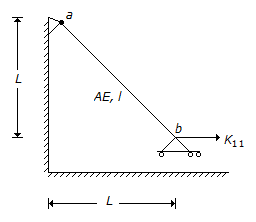# Civil Engineering - GATE Exam Questions

### Exercise :: GATE Exam Questions - Section 6

1.

A prestressed concrete rectangular beam of size 300 mm x 900 mm is prestressed with an initial prestressing force of 700 kN at an eccentricity of 350 mm at midspan. Stress at top of the beam due to prestress alone, in N/mm2 is

 A. 46 (tension) B. 2.59 (compression) C. zero D. 8.64 (compression)

Explanation:

No answer description available for this question. Let us discuss.

2.

In the plate bearing test, if the load applied is in the form of an inflated type of wheel, then this mechanism corresponds to :

 A. rigid plate B. flexible plate C. semi-rigid plate D. semi-elastic plate

Explanation:

No answer description available for this question. Let us discuss.

3.

Water table drops by 3 m in an irrigable land of 50 hectare. If porosity and specific retention are 0.30 and 0.10 respectively, the change in storage in hectare-meter is :

 A. 15 B. 30 C. 45 D. 60

Explanation:

No answer description available for this question. Let us discuss.

4.

Horizontal stiffness coefficient, K11 of bar ab is given by :A. A E/l 2 B. A E/2 1 C. A E/l D. 2 A E/l

Explanation:

No answer description available for this question. Let us discuss.

5.

The downstream end of a long prismatic channel of mild slope, ends in a pool created by a dam. The resulting non-uniform water surface profile can be described as one of the following :

 A. M3 profile ending in a hydraulic jump B. M1 profile that lies above normal depth line C. M2 profile that lies between critical and normal depth lines D. M3 profile that lies between critical and normal depth lines